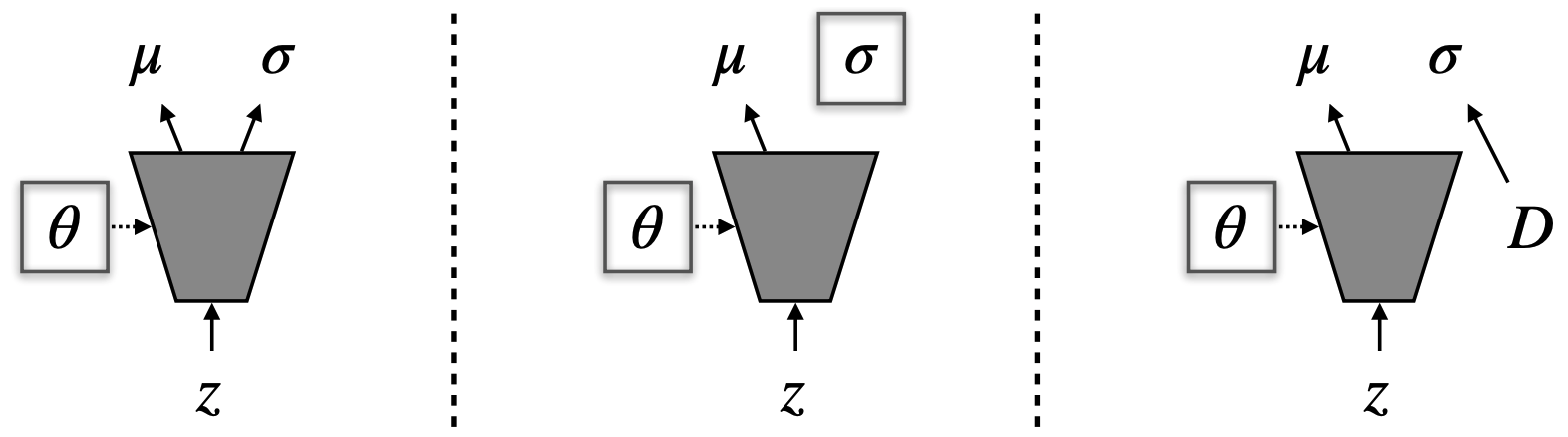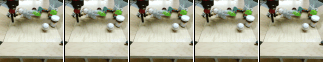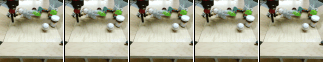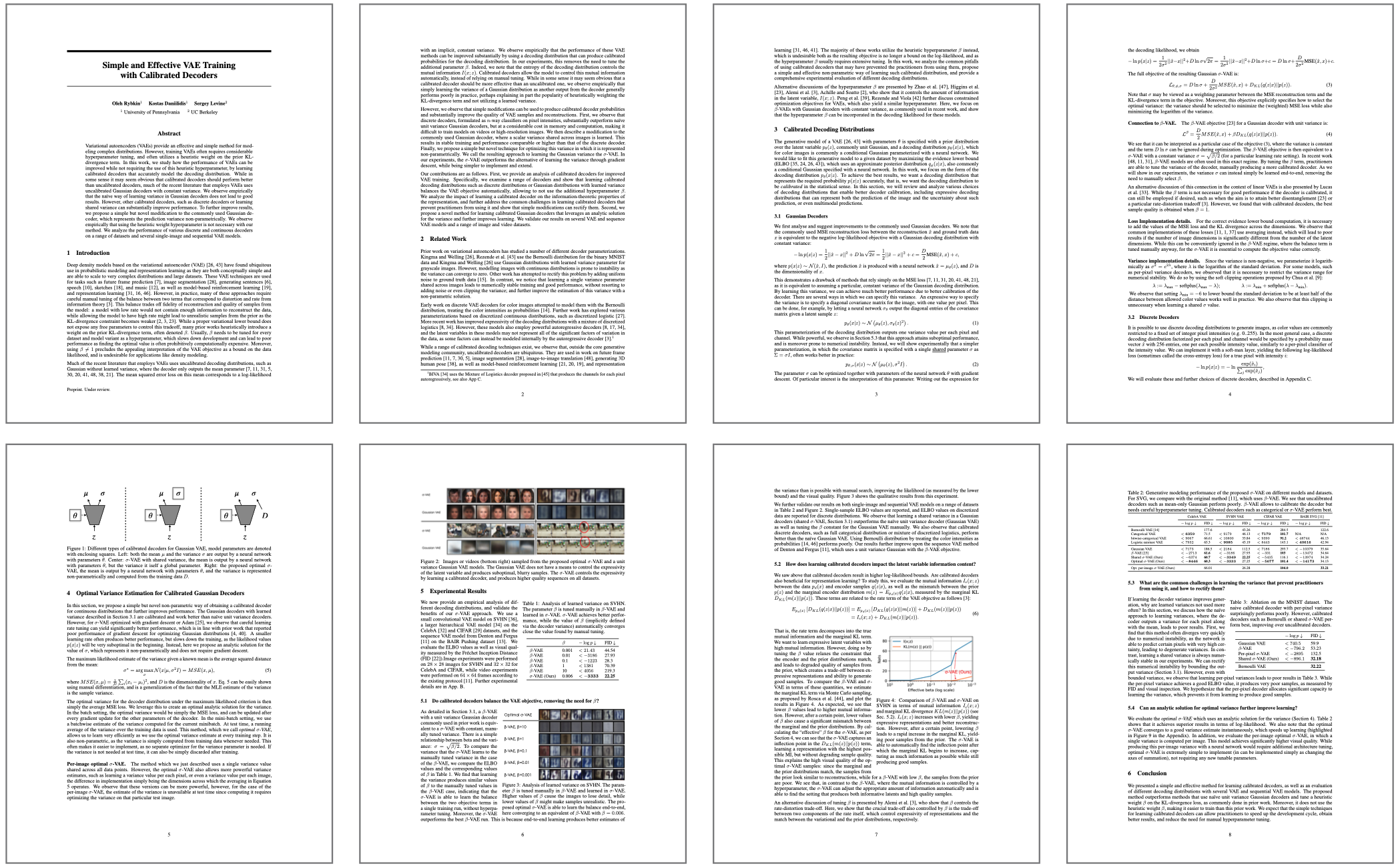Simple and Effective VAE Training with Calibrated Decoders
Simple and Effective VAE Training
with Calibrated Decoders
Oleh Rybkin
UPenn
Kostas Daniilidis
UPenn
Sergey Levine
UC Berkeley
International Conference on Machine Learning (ICML), 2021

Common implementations of VAE models, such as image VAEs or sequential video VAEs, use the MSE loss for reconstruction, and tune a heuristic weight on the KL divergence term of the objective. This MSE loss corresponds to a log-likelihood of a Gaussian decoder distribution with a certain constant variance. However, as we show in the paper, the assumption of constant variance is actually very problematic! Instead, it is better to learn the variance of the decoder, so that the decoding distribution is calibrated. We propose a simple method for learning such variance that empirically improves sample quality for multiple VAE and sequence VAE models. Moreover, we find that the weight on the KL divergence term can be simply set to one, as our method automatically balances the two terms of the objective. Our method improves performance over naive decoder choices, reduces the need for hyperparameter tuning, and can be implemented in 5 lines of code.

# σ-VAE

We note a correspondence between the hyperparameter β from β-VAE and the variance of the VAE decoder. By learning the variance of the decoder with ELBO, which we call σ-VAE, we can automatically train the balance weight between the two objective to be the optimal weight, producing good samples and avoiding extra hypeparameter tuning.

There are several design choices for learning the variance. The naive way would be to output a per pixel variance as another channel of the decoder (left). However, we empirically find that this often gives suboptimal results. Instead, learning a shared variance that is independent of the latent (center) often works better. We can further improve the optimization of this shared variance through an analytic solution (right). We call the resulting method σ-VAE.# Video Samples

### Gaussian VAE### σ-VAEA typical Gausssian VAE with unit variance (left) does not control the balance between the reconstruction and the KL-divergence loss and produces suboptimal, blurry samples. σ-VAE automatically balances the objective through learning the variance, which acts as a balance factor on the loss.

# Source Code

We released minimalistic implementations of the method in PyTorch and TensorFlow. It only takes 5 lines of code to try σ-VAE with your VAE model! We have also released an implementation of σ-VAE with the Stochastic Video Generation (SVG) method.

# Paper and BibtexCitation  Oleh Rybkin, Kostas Daniilidis, Sergey Levine. Simple and Effective VAE Training with Calibrated Decoders. [Bibtex] ```@misc{rybkin2020sigmavae, title={Simple and Effective VAE Training with Calibrated Decoders}, author={Oleh Rybkin and Kostas Daniilidis and Sergey Levine}, year={2020}, }```